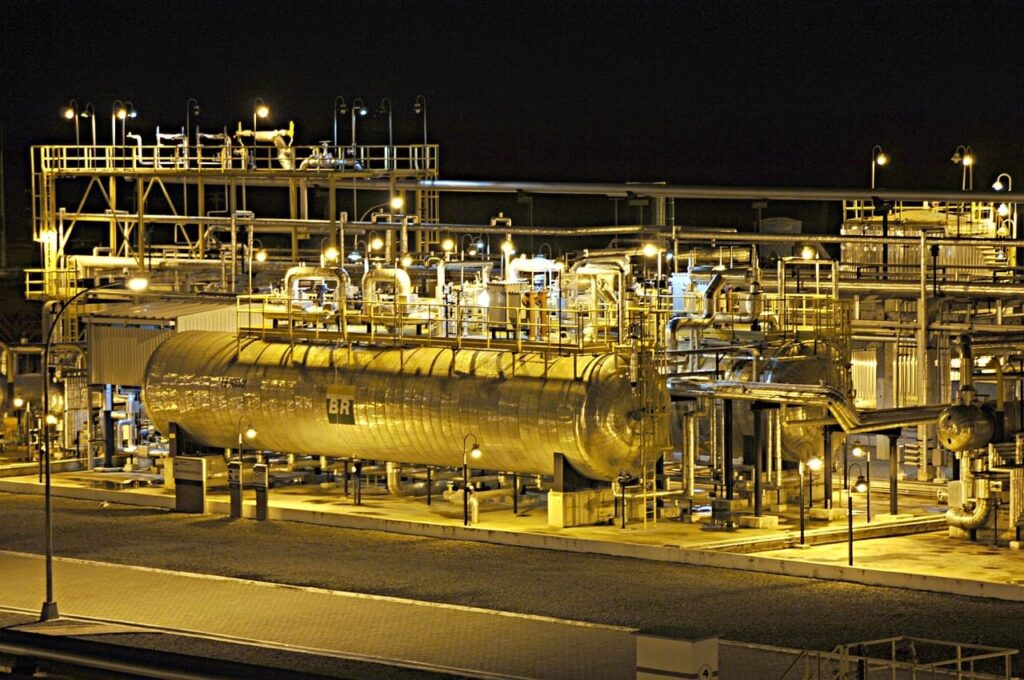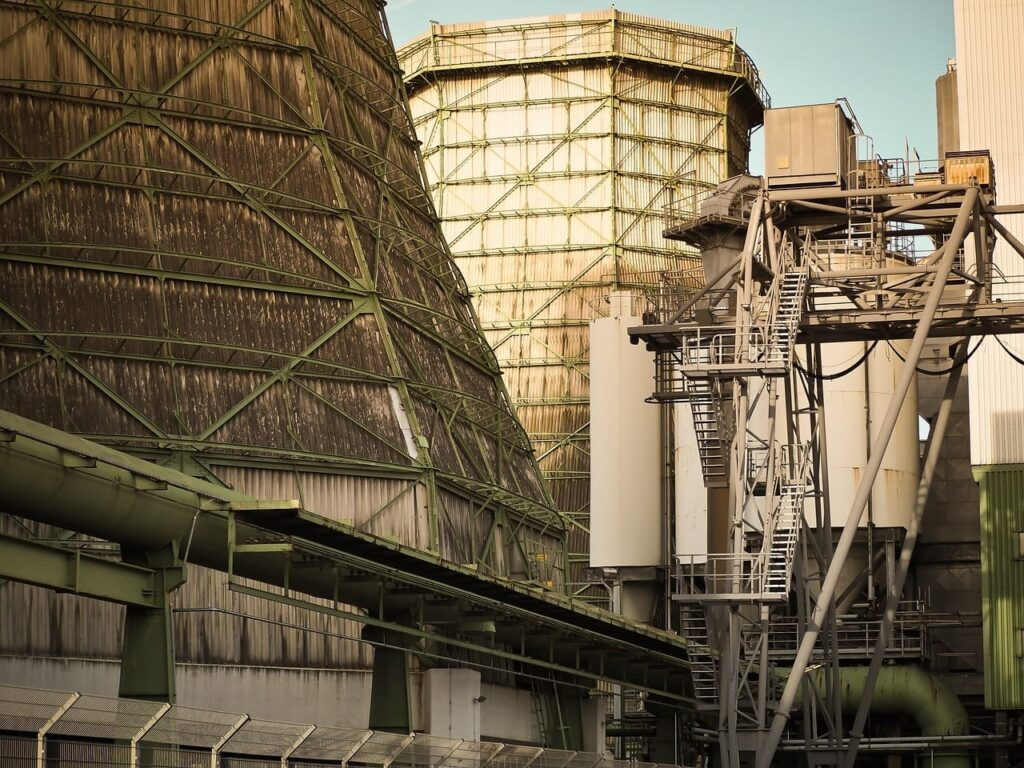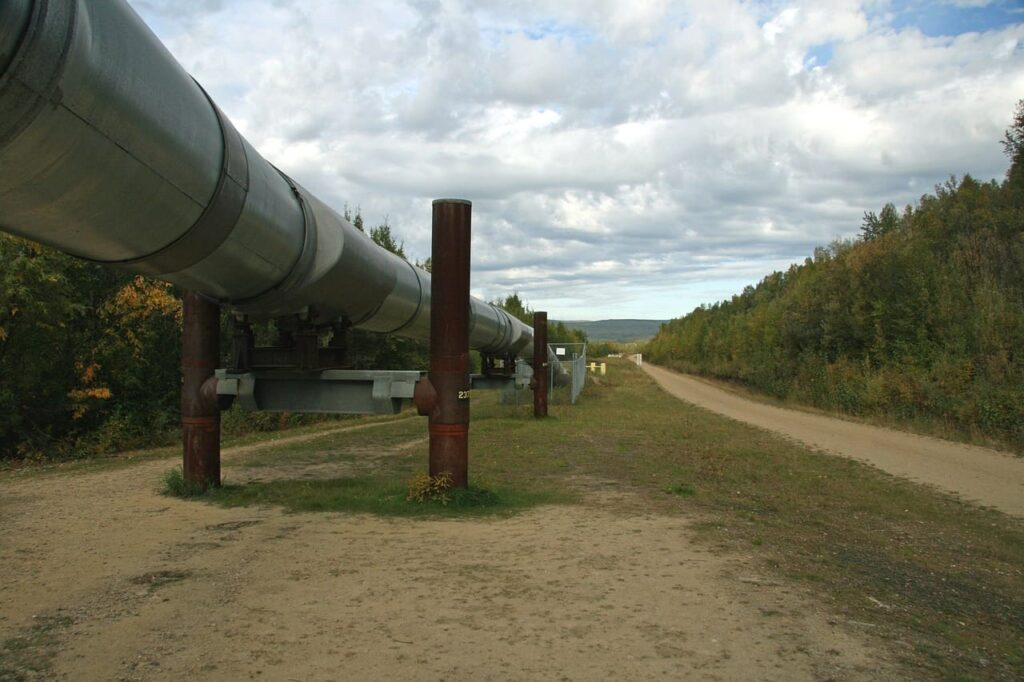# Power Engineering 101

Founder of Power Engineering 101. First Class Power Engineer. Empowering Power Engineers to achieve success on their SOPEEC exams.## How To: Calculate The Thickness Of A Blank, Unstayed Dished Head

The topic we’re going to cover in this post involves determining the minimum required thickness of a dished head, when it is a blank unstayed dished head with pressure on the concave side, and a segment of a sphere. We will review the formula, provide where to find it, define each of the variables, and then provide …## How To: Calculate The Maximum Allowable Working Pressure Of A Header

When completing ASME code calculations involving cylindrical components you will be required to know how to calculate the maximum allowable working pressure of a header. All page numbers referenced below are from the 2007 ASME Boiler & Pressure Vessel Code. Formula To calculate the maximum allowable working pressure of a header during different operating conditions you …## How To: Calculate The Minimum Required Thickness Of A Header

Throughout your 3rd Class Power Engineering studies you’ll be required to complete ASME code calculations involving cylindrical components. More specifically, you’ll be required to know how to calculate the minimum required thickness of a header on your provincial examination.   All page numbers referenced below are from the 2007 ASME Boiler & Pressure Vessel Code. Formula To …## How To: Calculate The Maximum Allowable Working Pressure Of A Boiler Drum

While completing ASME code calculations involving cylindrical components for 3rd Class Power Engineering you’ll be required to know how to calculate the maximum allowable working pressure of a boiler drum.  The referenced page numbers below are from the 2007 ASME Boiler & Pressure Vessel Code. Formula To calculate the maximum allowable working pressure of a …## How To: Calculate The Minimum Required Thickness Of A Boiler Drum

In 3rd Class Power Engineering ASME code calculations you’ll be required to know how to calculate the minimum required thickness of a boiler drum. The information provided below helps explain the material covered in:   All referenced page numbers below are from the 2007 ASME Boiler & Pressure Vessel Code. Formula To calculate the minimum …## How To: Calculate The Maximum Allowable Working Pressure Of Piping

While preparing for your 3A2 exam you’ll be required to complete ASME code calculations involving how to calculate the maximum allowable working pressure of piping. If you read through the material below. Complete the practice questions provided. You’ll have a much better understanding of the information covered within:  All page numbers referenced below are from …## How To: Calculate The Minimum Required Thickness Of Piping

While completing 3rd Class ASME code calculations involving cylindrical components you’ll be required to know how to calculate the minimum required thickness of piping. The objective of this post is to provide you with a clear understanding of how to accomplish this and explain the material covered in:   All page numbers referenced below are …